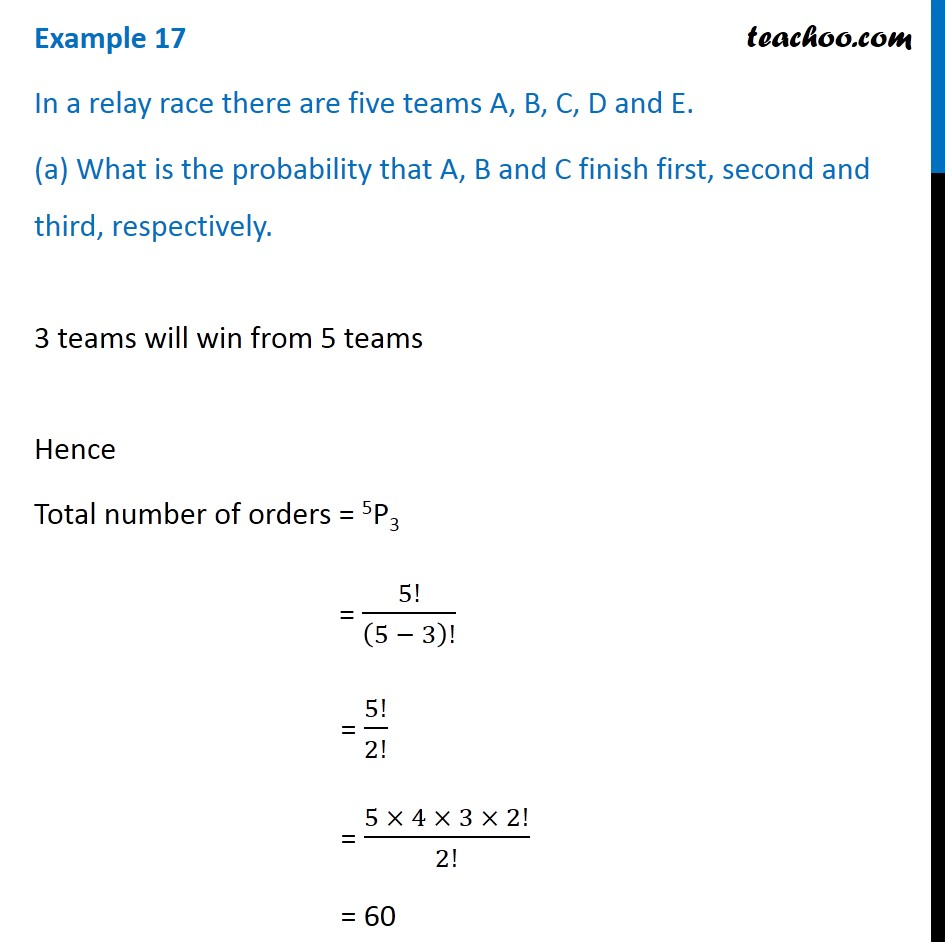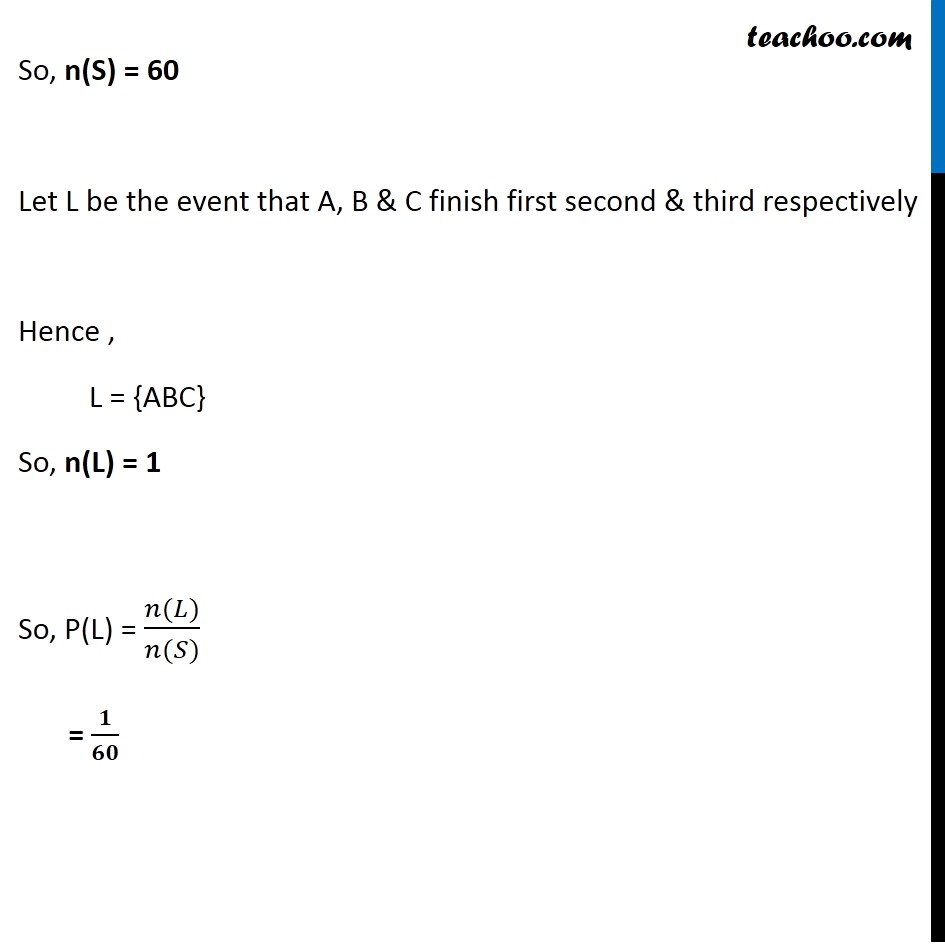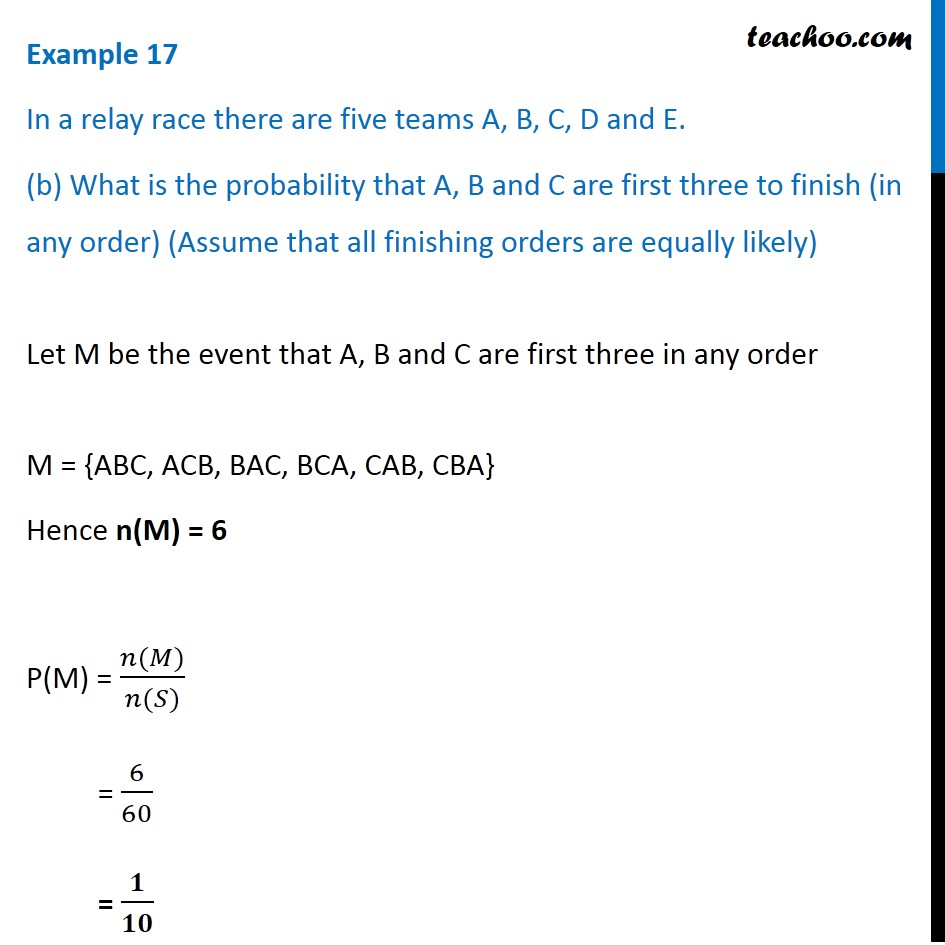Examples

Chapter 14 Class 11 Probability
Serial order wiseLearn in your speed, with individual attention - Teachoo Maths 1-on-1 Class

### Transcript

Example 17 In a relay race there are five teams A, B, C, D and E. (a) What is the probability that A, B and C finish first, second and third, respectively. 3 teams will win from 5 teams Hence Total number of orders = 5P3 = 5!/(5 − 3)! = 5!/2! = (5 × 4 × 3 × 2!)/2! = 60 So, n(S) = 60 Let L be the event that A, B & C finish first second & third respectively Hence , L = {ABC} So, n(L) = 1 So, P(L) = (𝑛(𝐿))/(𝑛(𝑆)) = 𝟏/𝟔𝟎 Example 17 In a relay race there are five teams A, B, C, D and E. (b) What is the probability that A, B and C are first three to finish (in any order) (Assume that all finishing orders are equally likely) Let M be the event that A, B and C are first three in any order M = {ABC, ACB, BAC, BCA, CAB, CBA} Hence n(M) = 6 P(M) = (𝑛(𝑀))/(𝑛(𝑆)) = 6/60 = 𝟏/𝟏𝟎